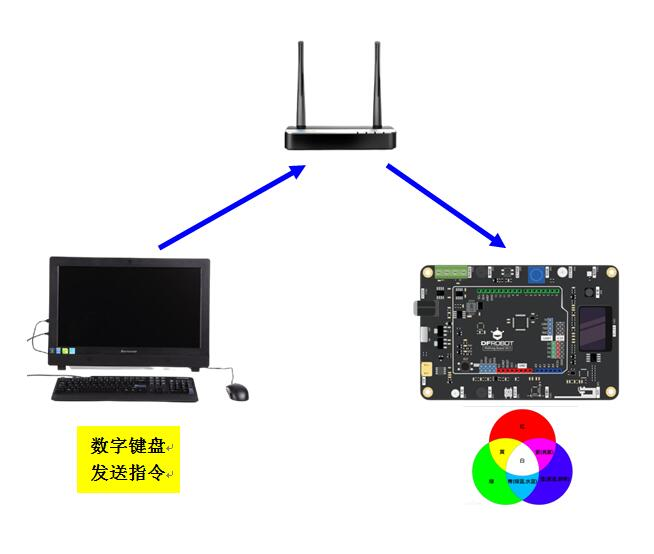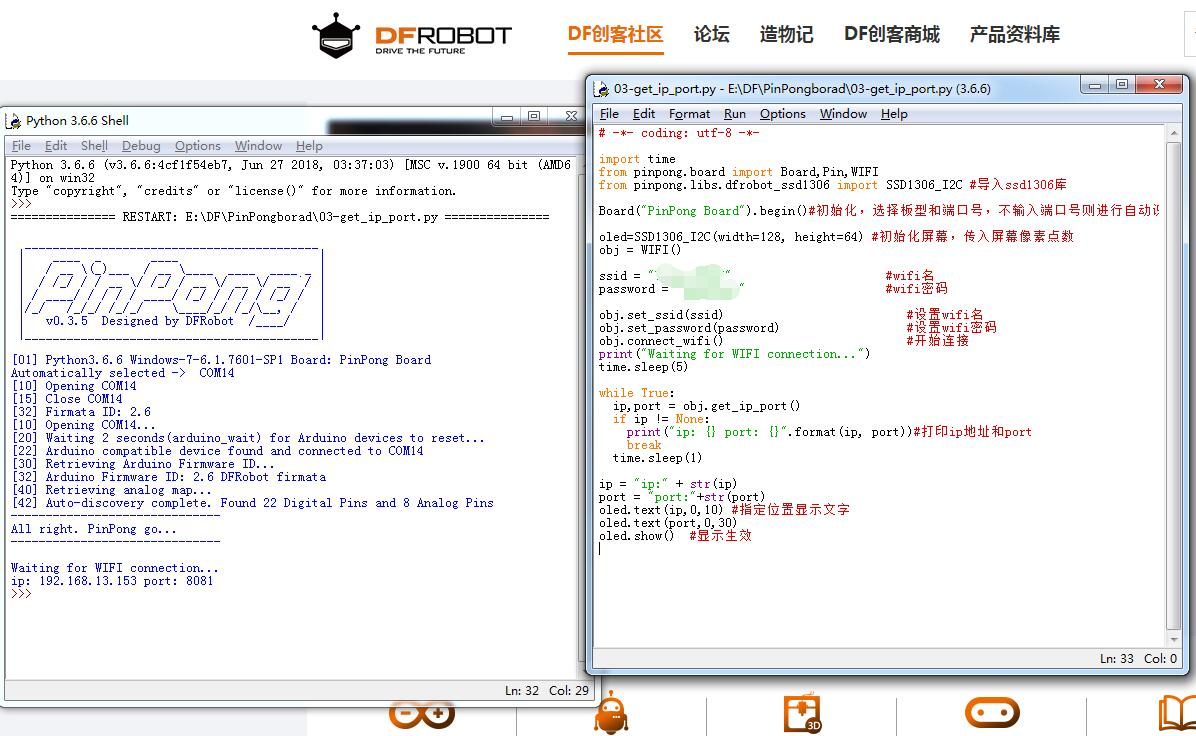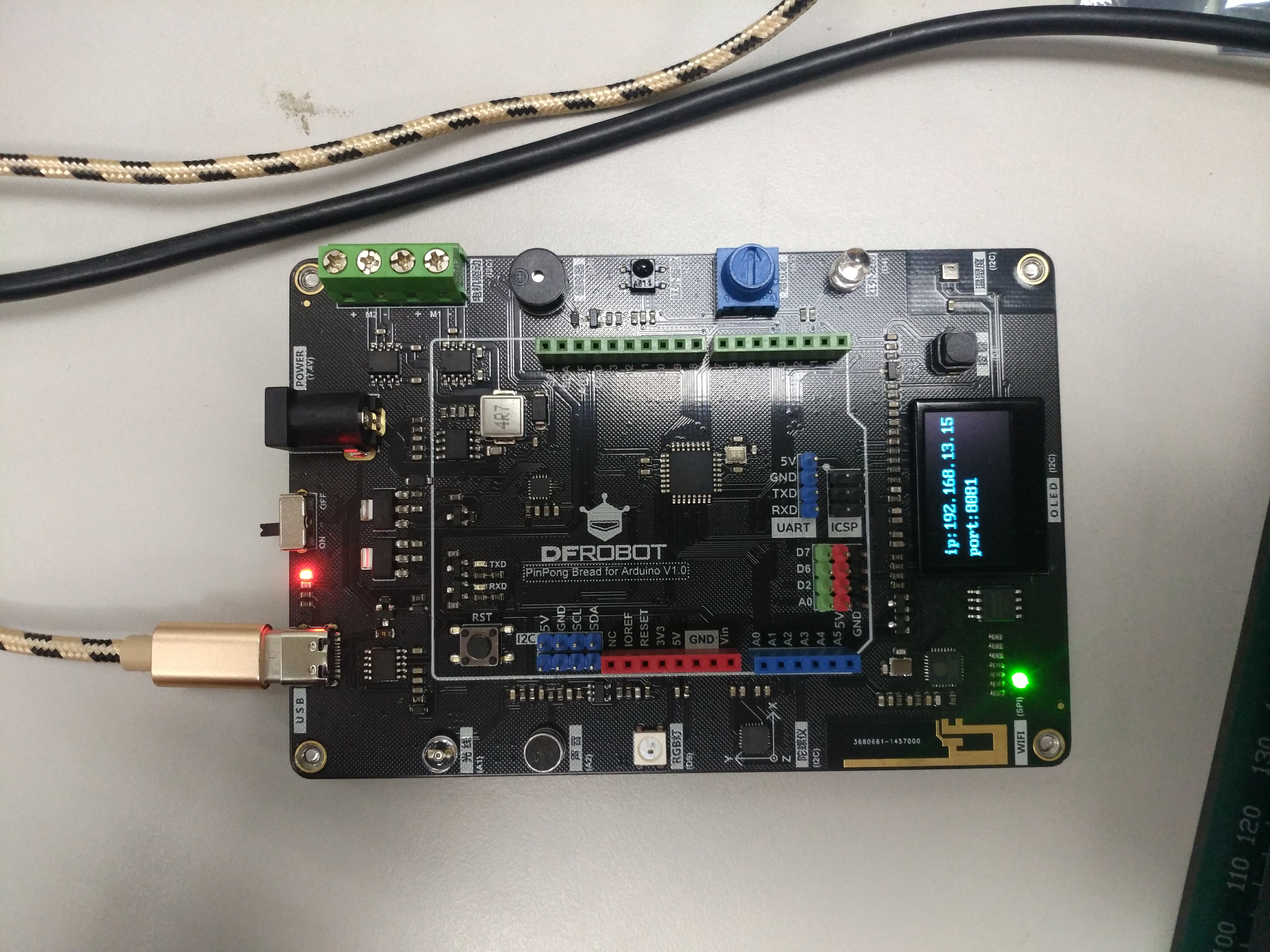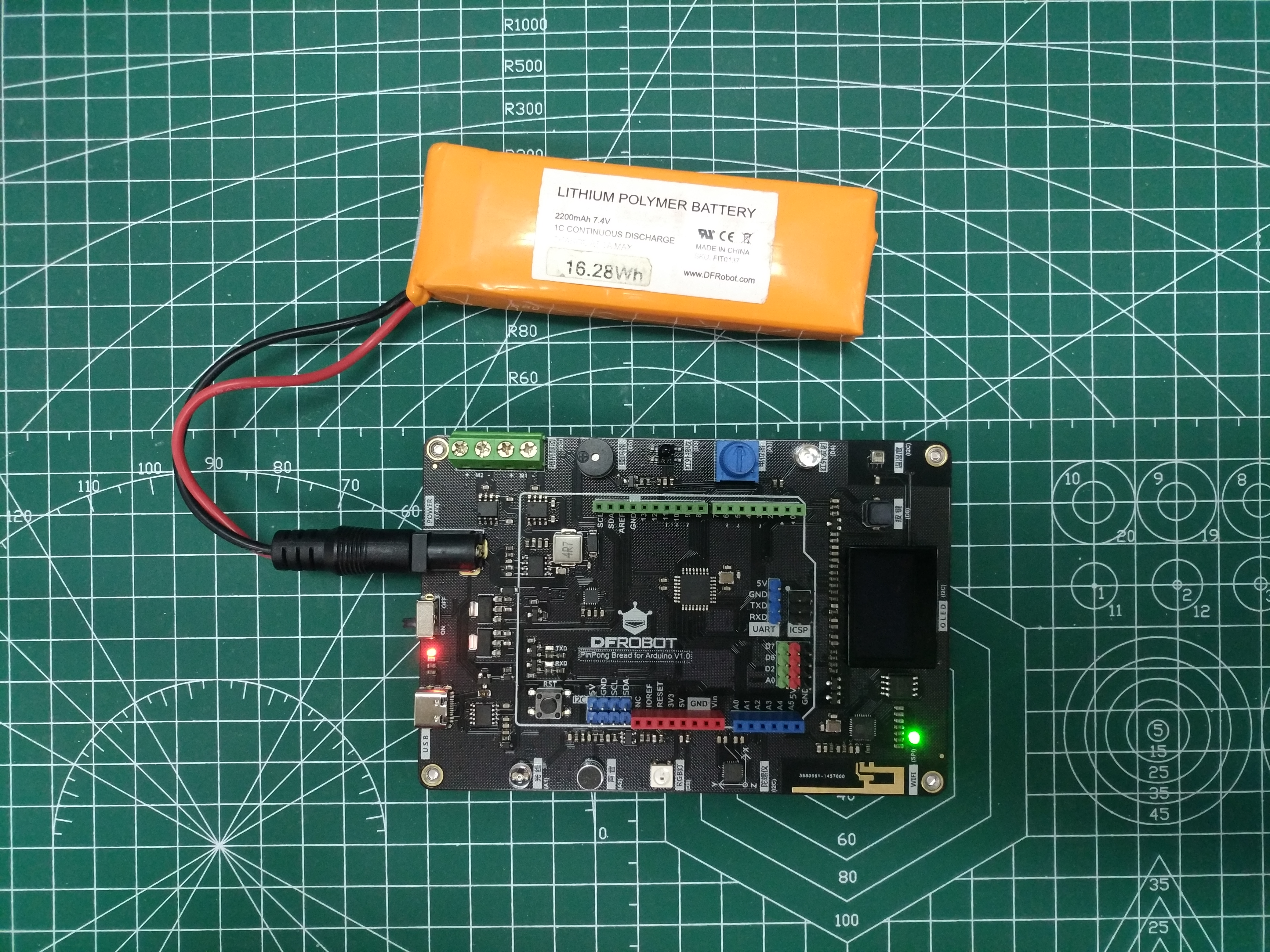[[d.project_title]][[d.material_name]]# 【PinPong Board】PinPong Board 玩物联2-物联RGB灯

#### 实验任务

1.掌握python缩进规则和单分支语句

2. 电脑数字键盘通过网络发送指令，经路由器传递，PinPong Board 上的RGB彩灯显示不同颜色1x
1x
7.4V 2500MA 锂电池 （带充放电保护板）
1x

1x

#### 1.什么是RGB LED灯？

RGB灯是以三原色共同交集成像，此外，也有蓝光LED配合黄色荧光 粉，以及紫外LED配合RGB荧光粉，整体来说，这两种都有其成像原理。目测测试板的是贴片RGB灯。

#### 3.Python知识点1---缩进规则

Python 采用代码缩进和冒号（ : ）来区分代码块之间的层次。

#### 4.Python知识点2---单分支if语句(流程图附后)

if(条件表达式)：

语句A

语句B```	                    					```# -*- coding: utf-8 -*-

import time
from pinpong.board import Board,Pin,WIFI
from pinpong.libs.dfrobot_ssd1306 import SSD1306_I2C #导入ssd1306库

Board("PinPong Board").begin()#初始化，选择板型和端口号，不输入端口号则进行自动识别

oled=SSD1306_I2C(width=128, height=64) #初始化屏幕，传入屏幕像素点数
obj = WIFI()

ssid = "test"                      #wifi名

obj.set_ssid(ssid)                          #设置wifi名
obj.connect_wifi()                          #开始连接
print("Waiting for WIFI connection...")
time.sleep(5)

while True:
ip,port = obj.get_ip_port()
if ip != None:
print("ip: {} port: {}".format(ip, port))#打印ip地址和port
break
time.sleep(1)

ip = "ip:" + str(ip)
port = "port:"+str(port)
oled.text(ip,0,10) #指定位置显示文字
oled.text(port,0,30)
oled.show()  #显示生效```
``````	                    					```#实验效果：根据输入序号RGB灯亮不同颜色
#接线：使用7.4V电池直接给PinPong主板供电，运行前打开主板的开关

import time
from pinpong.board import Board,Pin,NeoPixel

ip = "192.168.13.153"  #网络配置中OLEB屏上显示的ip
port = 8081    #网络配置中OLEB屏上显示的port

NEOPIXEL_PIN = Pin.D9
PIXELS_NUM = 1 #灯数
Board(ip, port)

np = NeoPixel(Pin(NEOPIXEL_PIN), PIXELS_NUM)

while True:
a=input('请输入序号')
if a=='1':
np = (255, 0 ,0)
if a=='2':
np = (0, 255 ,0)
if a=='3':
np = (0, 0 ,255)
if a=='4':
np = (255, 255,0)
if a=='5':
np = (0, 255 ,255)
if a=='6':
np = (255, 0 ,255)
if a=='7':
np = (255, 255 ,255)
if a=='8':
np = (0, 0 ,0)  ```
```

```	                    					```#实验效果：根据输入序号RGB灯亮不同颜色
#接线：使用7.4V电池直接给PinPong主板供电，运行前打开主板的开关

import time
from pinpong.board import Board,Pin,NeoPixel

ip = "192.168.13.153"  #网络配置中OLEB屏上显示的ip
port = 8081    #网络配置中OLEB屏上显示的port

NEOPIXEL_PIN = Pin.D9
PIXELS_NUM = 1 #灯数
Board(ip, port)

np = NeoPixel(Pin(NEOPIXEL_PIN), PIXELS_NUM)

while True:
a=input('请输入序号')
if a=='1': np = (255, 0 ,0)
if a=='2': np = (0, 255 ,0)
if a=='3': np = (0, 0 ,255)
if a=='4': np = (255, 255,0)
if a=='5': np = (0, 255 ,255)
if a=='6': np = (255, 0 ,255)
if a=='7': np = (255, 255 ,255)
if a=='8': np = (0, 0 ,0)   ```
```

RGB配色参考

Makelog作者原创文章，未经授权禁止转载。
0
0[[c.user_name]] [[c.create_time]]
[[c.parent_comment.count]]
|
[[c.comment_content]]
[[r.user.user_name]] [[r.create_time]]
[[r.comment_content]]

0
4
2
8
14
33
5
25
2
5